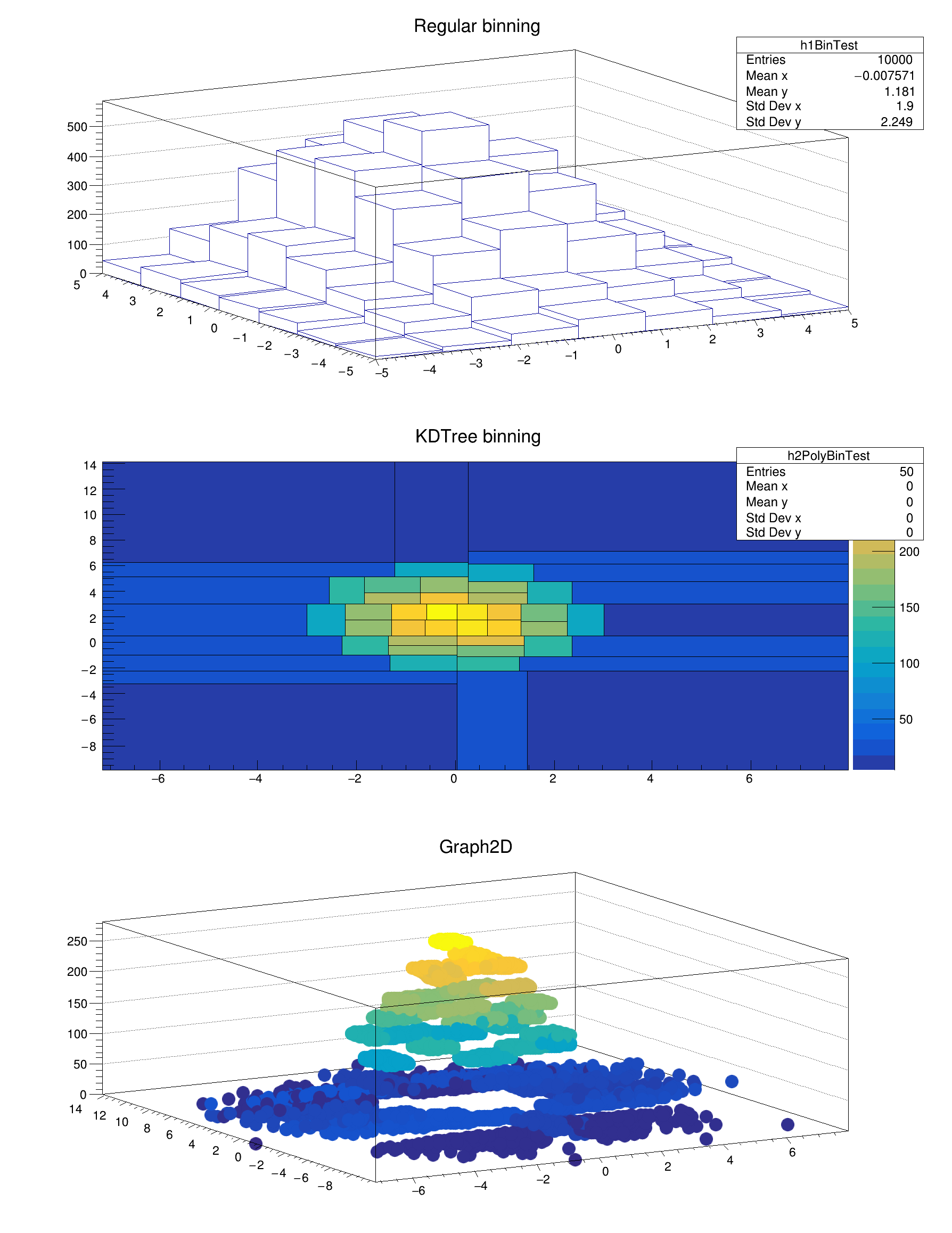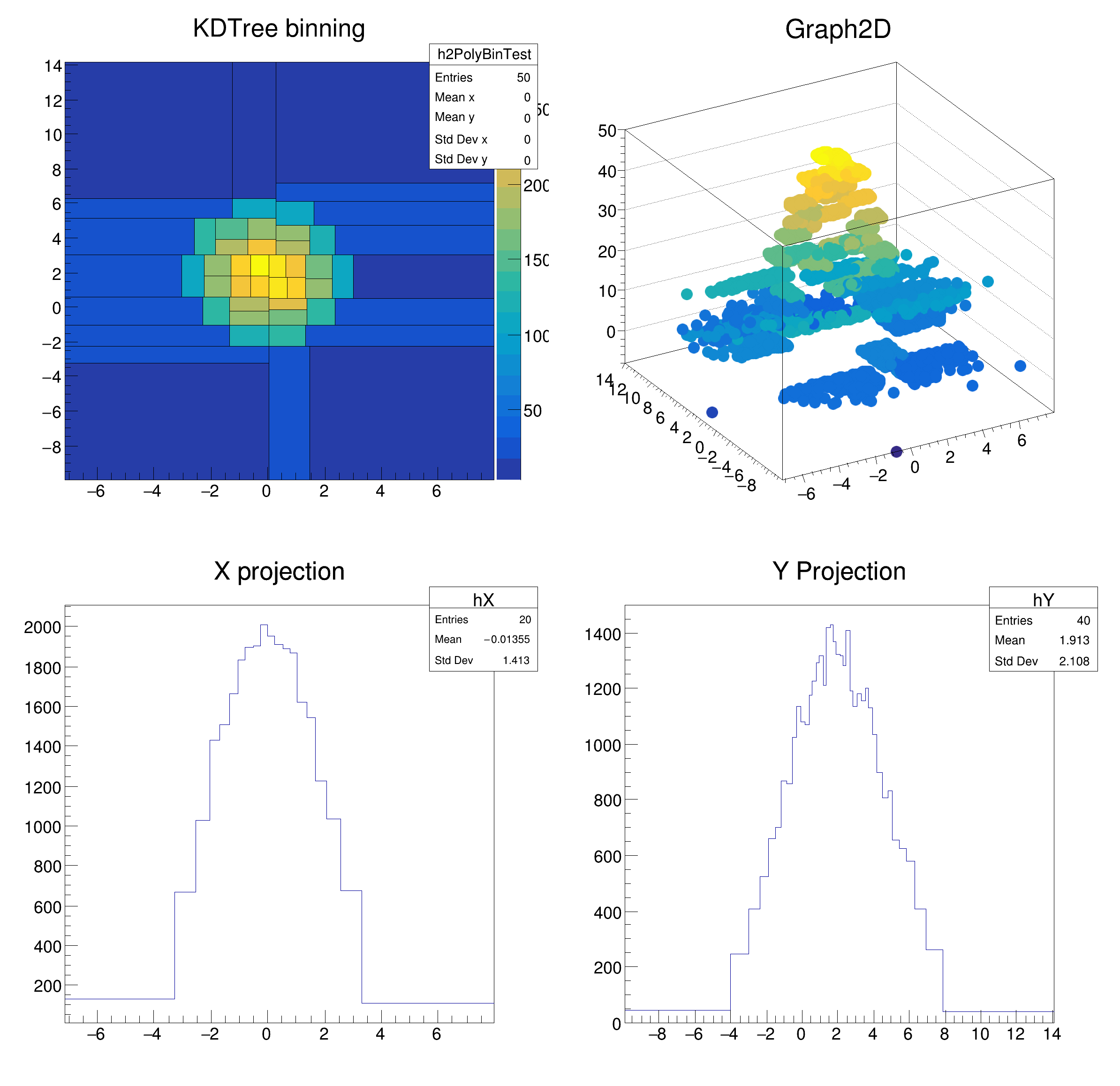ROOT   Reference Guide
Searching...
No Matches
kdTreeBinning.C File Reference

## Detailed DescriptionkdTreeBinning tutorial: bin the data in cells of equal content using a kd-tree

Using TKDTree wrapper class as a data binning structure Plot the 2D data using the TH2Poly classBin with minimum density: 13
Bin with maximum density: 29
Bin with minimum density: 0
Bin with maximum density: 49
#include <math.h>
#include "TKDTreeBinning.h"
#include "TH2D.h"
#include "TH2Poly.h"
#include "TStyle.h"
#include "TGraph2D.h"
#include "TRandom3.h"
#include "TCanvas.h"
#include <iostream>
void kdTreeBinning() {
// -----------------------------------------------------------------------------------------------
// Create random sample with regular binning plotting
const UInt_t DATASZ = 10000;
const UInt_t NBINS = 50;
double mu = {0,2};
double sig = {2,3};
r.SetSeed(1);
for (UInt_t i = 0; i < DATADIM; ++i)
for (UInt_t j = 0; j < DATASZ; ++j)
smp[DATASZ * i + j] = r.Gaus(mu[i], sig[i]);
UInt_t h1bins = (UInt_t) sqrt(NBINS);
TH2D* h1 = new TH2D("h1BinTest", "Regular binning", h1bins, -5., 5., h1bins, -5., 5.);
for (UInt_t j = 0; j < DATASZ; ++j)
h1->Fill(smp[j], smp[DATASZ + j]);
// ---------------------------------------------------------------------------------------------
// Create KDTreeBinning object with TH2Poly plotting
TKDTreeBinning* kdBins = new TKDTreeBinning(DATASZ, DATADIM, smp, NBINS);
UInt_t nbins = kdBins->GetNBins();
UInt_t dim = kdBins->GetDim();
const Double_t* binsMinEdges = kdBins->GetBinsMinEdges();
const Double_t* binsMaxEdges = kdBins->GetBinsMaxEdges();
TH2Poly* h2pol = new TH2Poly("h2PolyBinTest", "KDTree binning", kdBins->GetDataMin(0), kdBins->GetDataMax(0), kdBins->GetDataMin(1), kdBins->GetDataMax(1));
for (UInt_t i = 0; i < nbins; ++i) {
UInt_t edgeDim = i * dim;
h2pol->AddBin(binsMinEdges[edgeDim], binsMinEdges[edgeDim + 1], binsMaxEdges[edgeDim], binsMaxEdges[edgeDim + 1]);
}
for (UInt_t i = 1; i <= kdBins->GetNBins(); ++i)
h2pol->SetBinContent(i, kdBins->GetBinDensity(i - 1));
std::cout << "Bin with minimum density: " << kdBins->GetBinMinDensity() << std::endl;
std::cout << "Bin with maximum density: " << kdBins->GetBinMaxDensity() << std::endl;
TCanvas* c1 = new TCanvas("glc1", "TH2Poly from a kdTree",0,0,600,800);
c1->Divide(1,3);
c1->cd(1);
h1->Draw("lego");
c1->cd(2);
h2pol->Draw("COLZ L");
c1->Update();
//-------------------------------------------------
// Draw an equivalent plot showing the data points
std::vector<Double_t> z = std::vector<Double_t>(DATASZ, 0.);
for (UInt_t i = 0; i < DATASZ; ++i)
z[i] = (Double_t) h2pol->GetBinContent(h2pol->FindBin(smp[i], smp[DATASZ + i]));
TGraph2D *g = new TGraph2D(DATASZ, smp, &smp[DATASZ], &z);
g->SetMarkerStyle(20);
c1->cd(3);
g->Draw("pcol");
c1->Update();
// ---------------------------------------------------------
// make a new TH2Poly where bins are ordered by the density
TH2Poly* h2polrebin = new TH2Poly("h2PolyBinTest", "KDTree binning", kdBins->GetDataMin(0), kdBins->GetDataMax(0), kdBins->GetDataMin(1), kdBins->GetDataMax(1));
h2polrebin->SetFloat();
//---------------------------------
// Sort the bins by their density
kdBins->SortBinsByDensity();
for (UInt_t i = 0; i < kdBins->GetNBins(); ++i) {
const Double_t* binMinEdges = kdBins->GetBinMinEdges(i);
const Double_t* binMaxEdges = kdBins->GetBinMaxEdges(i);
}
for (UInt_t i = 1; i <= kdBins->GetNBins(); ++i){
h2polrebin->SetBinContent(i, kdBins->GetBinDensity(i - 1));}
std::cout << "Bin with minimum density: " << kdBins->GetBinMinDensity() << std::endl;
std::cout << "Bin with maximum density: " << kdBins->GetBinMaxDensity() << std::endl;
// now make a vector with bin number vs position
for (UInt_t i = 0; i < DATASZ; ++i)
z[i] = (Double_t) h2polrebin->FindBin(smp[i], smp[DATASZ + i]);
TGraph2D *g2 = new TGraph2D(DATASZ, smp, &smp[DATASZ], &z);
g2->SetMarkerStyle(20);
// plot new TH2Poly (ordered one) and TGraph2D
// The new TH2Poly has to be same as old one and the TGraph2D should be similar to
// the previous one. It is now made using as z value the bin number
TCanvas* c4 = new TCanvas("glc4", "TH2Poly from a kdTree (Ordered)",50,50,800,800);
c4->Divide(2,2);
c4->cd(1);
h2polrebin->Draw("COLZ L"); // draw as scatter plot
c4->cd(2);
g2->Draw("pcol");
c4->Update();
// make also the 1D binned histograms
TKDTreeBinning* kdX = new TKDTreeBinning(DATASZ, 1, &smp, 20);
TKDTreeBinning* kdY = new TKDTreeBinning(DATASZ, 1, &smp[DATASZ], 40);
TH1* hX=new TH1F("hX", "X projection", kdX->GetNBins(), kdX->GetOneDimBinEdges());
for(int i=0; i<kdX->GetNBins(); ++i){
hX->SetBinContent(i+1, kdX->GetBinDensity(i));
}
TH1* hY=new TH1F("hY", "Y Projection", kdY->GetNBins(), kdY->GetOneDimBinEdges());
for(int i=0; i<kdY->GetNBins(); ++i){
hY->SetBinContent(i+1, kdY->GetBinDensity(i));
}
c4->cd(3);
hX->Draw();
c4->cd(4);
hY->Draw();
}
ROOT::R::TRInterface & r
Definition Object.C:4
#define g(i)
Definition RSha256.hxx:105
unsigned int UInt_t
Definition RtypesCore.h:46
double Double_t
Definition RtypesCore.h:59
double sqrt(double)
virtual void SetMarkerStyle(Style_t mstyle=1)
Set the marker style.
Definition TAttMarker.h:40
The Canvas class.
Definition TCanvas.h:23
Definition TCanvas.cxx:708
void Update() override
Definition TCanvas.cxx:2504
Graphics object made of three arrays X, Y and Z with the same number of points each.
Definition TGraph2D.h:41
virtual void Draw(Option_t *option="P0")
Specific drawing options can be used to paint a TGraph2D:
Definition TGraph2D.cxx:712
1-D histogram with a float per channel (see TH1 documentation)}
Definition TH1.h:575
TH1 is the base class of all histogram classes in ROOT.
Definition TH1.h:58
virtual Int_t Fill(Double_t x)
Increment bin with abscissa X by 1.
Definition TH1.cxx:3350
virtual void SetBinContent(Int_t bin, Double_t content)
Set bin content see convention for numbering bins in TH1::GetBin In case the bin number is greater th...
Definition TH1.cxx:9062
virtual void Draw(Option_t *option="")
Draw this histogram with options.
Definition TH1.cxx:3073
2-D histogram with a double per channel (see TH1 documentation)}
Definition TH2.h:292
2D Histogram with Polygonal Bins
Definition TH2Poly.h:66
void SetFloat(Bool_t flag=true)
When set to kTRUE, allows the histogram to expand if a bin outside the limits is added.
Definition TH2Poly.cxx:1297
virtual Double_t GetBinContent(Int_t bin) const
Returns the content of the input bin For the overflow/underflow/sea bins:
Definition TH2Poly.cxx:765
Adds a new bin to the histogram.
Definition TH2Poly.cxx:222
virtual void SetBinContent(Int_t bin, Double_t content)
Sets the contents of the input bin to the input content Negative values between -1 and -9 are for the...
Definition TH2Poly.cxx:1280
Int_t FindBin(Double_t x, Double_t y, Double_t z=0)
Returns the bin number of the bin at the given coordinate.
Definition TH2Poly.cxx:546
<- TKDTreeBinning - A class providing multidimensional binning ->
const Double_t * GetBinMaxEdges(UInt_t bin) const
Returns the bin's maximum edges. 'bin' is between 0 and fNBins - 1.
const Double_t * GetBinsMaxEdges() const
Returns an array with all bins' maximum edges The edges are arranges as xmax_1,ymax_1,...
UInt_t GetBinMaxDensity() const
Return the bin with maximum density.
UInt_t GetNBins() const
Returns the number of bins.
Double_t GetDataMax(UInt_t dim) const
Returns the maximum of the data in the dim coordinate. 'dim' is between 0 and fDim - 1.
const Double_t * GetBinMinEdges(UInt_t bin) const
Returns the bin's minimum edges. 'bin' is between 0 and fNBins - 1.
Double_t GetDataMin(UInt_t dim) const
Returns the minimum of the data in the dim coordinate. 'dim' is between 0 and fDim - 1.
void SortBinsByDensity(Bool_t sortAsc=kTRUE)
Sorts bins by their density.
Double_t GetBinDensity(UInt_t bin) const
Returns the density in bin.
const Double_t * GetBinsMinEdges() const
Returns an array with all bins' minimum edges The edges are arranges as xmin_1,ymin_1,...
UInt_t GetBinMinDensity() const
Return the bin with minimum density.
const Double_t * GetOneDimBinEdges() const
Returns a pointer to the vector of the bin edges for one dimensional binning only.
UInt_t GetDim() const
Returns the number of dimensions.
const Double_t * SortOneDimBinEdges(Bool_t sortAsc=kTRUE)
Sort the one-dimensional bin edges and retuns a pointer to them.
void Divide(Int_t nx=1, Int_t ny=1, Float_t xmargin=0.01, Float_t ymargin=0.01, Int_t color=0) override